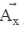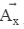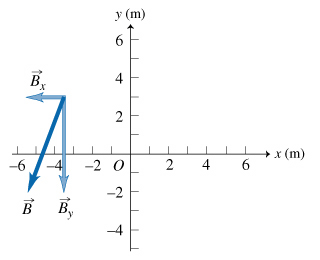# Problem: Tactics Box: Determining the components of a vector1. The absolute value |Ax| of the x component Ax is the magnitude of the component vector .2. The sign of Ax is positive if points in the positive x direction; it is negative if points in the negative x direction.3. The y component is determined similarly.Now combine the information given in the Tactics Box above to find the x and y components, Bx and By, of vector B? Shown in the figure.

###### FREE Expert Solution

Bx = Bhead - Btail = -5.5 - (-3.5) = - 2.0 m

|Bx| = 2 m

The magnitude of Bx is 2 m

95% (399 ratings)###### Problem Details

Tactics Box: Determining the components of a vector

1. The absolute value |Ax| of the x component Ax is the magnitude of the component vector.

2. The sign of Ax is positive ifpoints in the positive x direction; it is negative ifpoints in the negative x direction.

3. The y component is determined similarly.

Now combine the information given in the Tactics Box above to find the x and y components, Bx and By, of vector B? Shown in the figure.